• Matlab中创建一个矩阵的3种常用方法: 1、直接法：  A=[1 2 3;4 5 6]或A=[1,2,3;4,5,6]。  以上需注意两点，第一，矩阵a大小不用提前设定，Matlab根据后边矩阵内容自动确定。第二，矩阵同一行元素用空格' '或逗号'...

Matlab中创建一个矩阵的3种常用方法:

1、直接法：
A=[1 2 3;4 5 6]或A=[1,2,3;4,5,6]。
以上需注意两点，第一，矩阵a大小不用提前设定，Matlab根据后边矩阵内容自动确定。第二，矩阵同一行元素用空格' '或逗号','隔开，不同行用分号';'或回车分开。例如：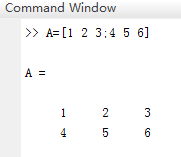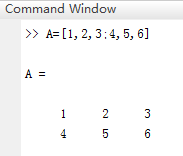2、冒号表达式法（一维）
B=a:h:b;  其中a,b代表一维矩阵（向量）的区间，h为步宽，例如：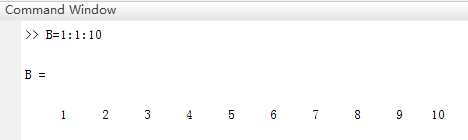需注意，b值有可能取不到，因为矩阵是以a为首元素，按步宽前进。

3、函数法(一维)
linspace(a,b,n)；其中a,b分别为一维矩阵（向量）的首末元素，n为总元素数（默认为100），例如：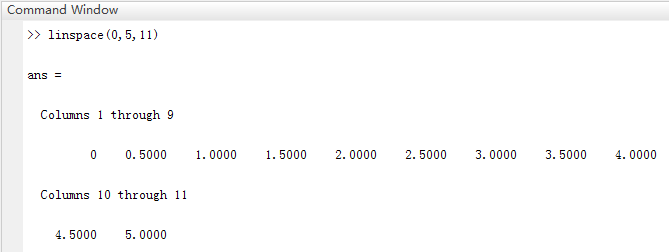以上是三种常用的自定义矩阵方法，自己也可以运用Matlab提供的函数创建特殊函数。

展开全文• 矩阵的元素之间用 空格 或 逗号 分隔 矩阵行与行之间用 分号 分开 直接输入法，分号可以用 回车 代替 A=[1 2 3 4 5 6 7 8 9;1 2 3] 利用MATLAB函数创建矩阵 基本矩阵函数如下： (1) ones()函数：产生全为...
矩阵定义
直接输入法
A=[1 2 3;4 5 6;7 8 9]

矩阵用方括号 “[ ]” 括起
矩阵同一行中的元素之间用 空格 或 逗号 分隔
矩阵行与行之间用 分号  分开
直接输入法中，分号可以用 回车 代替
A=[1 2 3
4 5 6
7 8 9;1 2 3]

由向量生成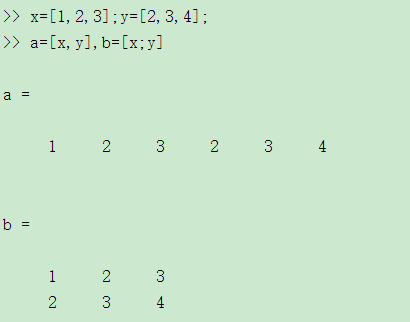通过编写m文件生成
利用MATLAB函数创建矩阵
基本矩阵函数如下：
(1) ones()函数：产生全为1的矩阵，ones(n)：产生nn维的全1矩阵，ones(m,n)：产生mn维的全1矩阵；
(2) zeros()函数：产生全为0的矩阵；
(3) rand()函数：产生在（0，1）区间均匀分布的随机阵；
(4) eye()函数：产生单位阵；
(5) randn()函数：产生均值为0，方差为1的标准正态分布随机矩阵。
(6)tril()下三角矩阵
(7)triu()上三角矩阵
(8)diag（X）：若 X 是矩阵，则 diag(X) 为 X 的主对角线向量
若 X 是向量，diag(X) 产生以 X 为主对角线的对角矩阵
diag(X,k)是提取第k条对角线的元素
使用格式
A=zeros(N) 产生NN的全零矩阵
A=zeros(M,N) 产生MN的全零矩阵
A=zeros(M,N,P,…)产生MNP*…的全零矩阵
A=zeros(siza(b)) 产生和矩阵B维数相同的全零矩阵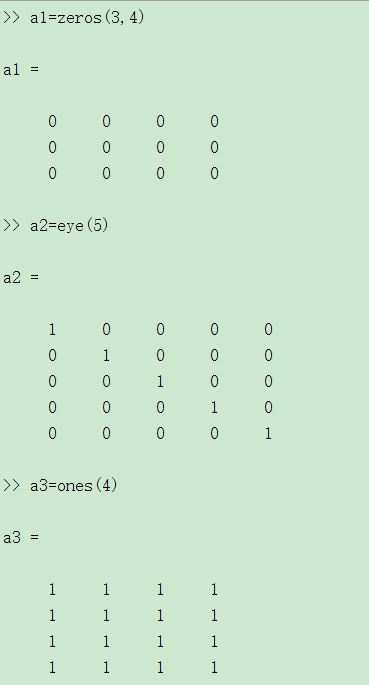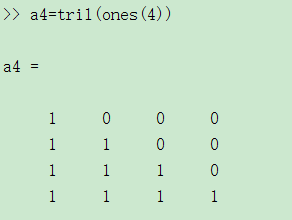生成五阶的随机矩阵，元素在10到90之间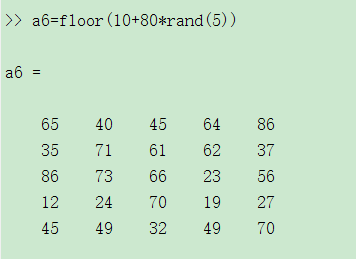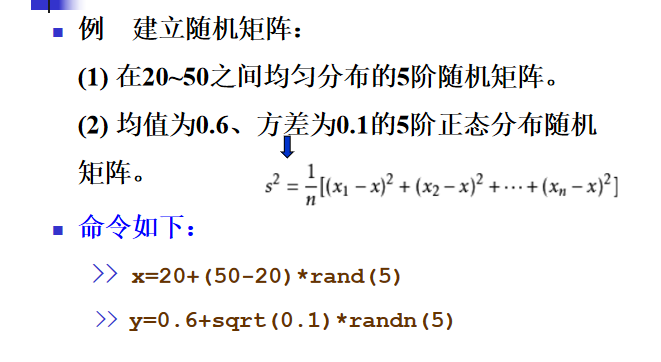矩阵元素赋值
矩阵元素可以是任何形式的表达
x=[-1.3,sqrt(3),(1+2+3)*4/5]

矩阵元素的单独赋值
x(5)=abs(x(1))

Matlab自动将向量 x 的长度扩展到 5，
并将未赋值部分置零。
大矩阵可以把小矩阵作为其元素
A=[A;11 12 13]

矩阵元素的引用
单个元素的引用
x(i)向量x中的第i个元素
A(i,j)矩阵A中的第i行，第j列元素
多个元素的引用：冒号的特殊用法
产生一个由等差序列组成的向量； a 是首项，b 是公差，c 确定最后一项；若 b=1，则 b 可以省略。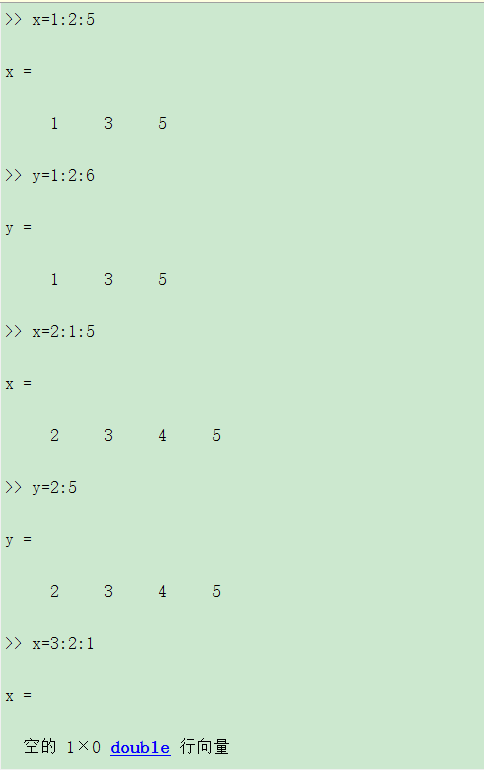A(i:j, m:n) 表示由矩阵 A 的第 i 到第 j 行和第 m 到第 n
列交叉线上的元素组成的子矩阵。
可利用冒号提取矩阵 的整行或整列。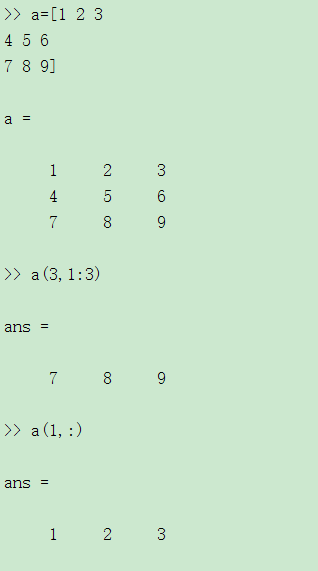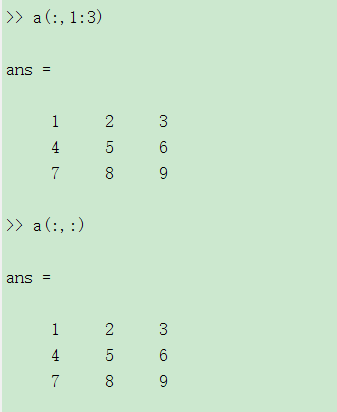用于专门学科的特殊矩阵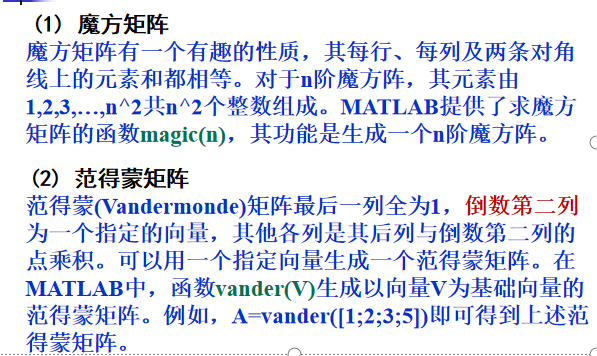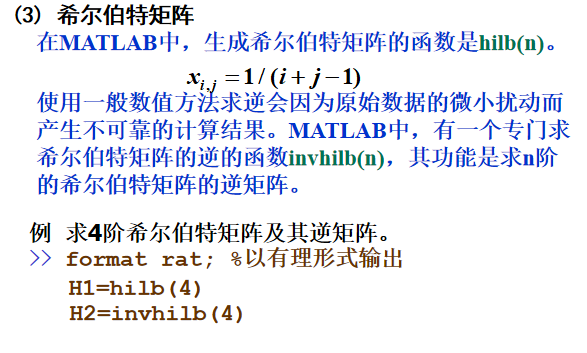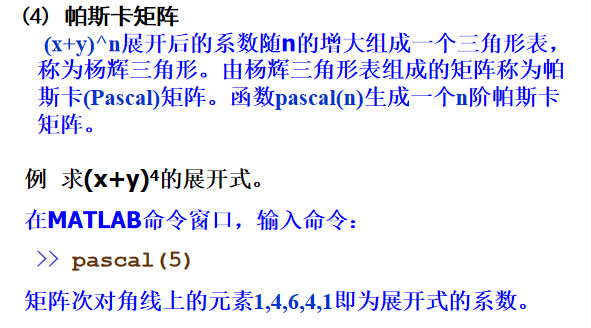矩阵操作
提取矩阵的部分元素： 冒号运算符

A( : )
A的所有元素

A(: , : )
二维矩阵A的所有元素

A(:,k)
A的第K列

A（k,:）
A的第k行

A（k:m）
A的第k个元素到第m个元素

A(:,k:m)
A的第 k 到第 m 列组成的子矩阵

矩阵的旋转

fliplr(A)
左右翻转

flipud(A)
上下翻转

rot90(A)
逆时针旋转90度

rot90(A,k)
逆时针旋转k*90度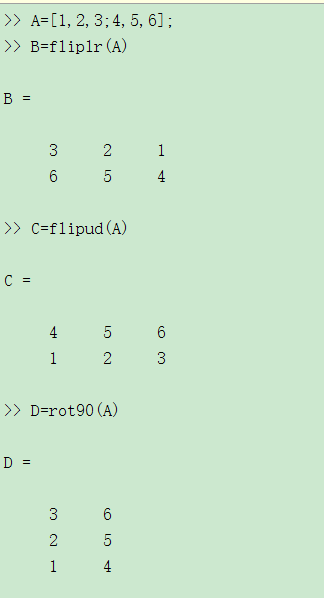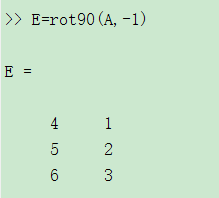矩阵转置与共轭转置
把矩阵A的行和列互相交换所产生的矩阵称为A的转置矩阵，这一过程称为矩阵的转置。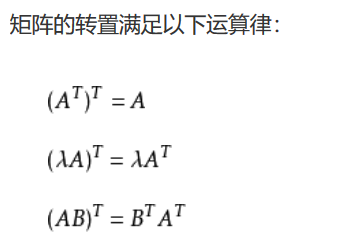矩阵 A 的共轭转置A * 定义为:将矩阵A 的行与列对换，且在将行与列对换时还要将每个元素换成该元素的共轭。

’
共轭转置

.’
转置，矩阵元素不能取共轭

点与单引号之间不能有空格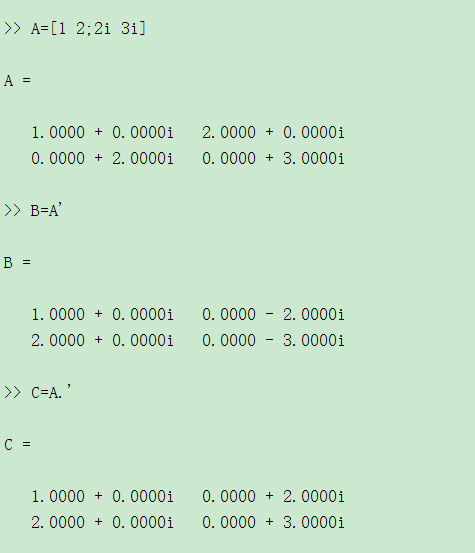矩阵的逆和伪逆
对于一个方阵A,如果存在一个与之同阶方针B，使得AB=BA=E,此时矩阵B为A的逆矩阵，A也是B的逆矩阵，使用函数inv(A)
矩阵的伪逆如果矩阵A不是一个方阵，或者A是一个非满秩的方阵时，矩阵A没有逆矩阵，但可以找到一个与A的转置矩阵A’同型的矩阵B，使得：ABA=A，BAB=B 此时称矩阵B为矩阵A的伪逆，也称为广义逆矩阵。在MATLAB中，求一个矩阵伪逆的函数是pinv(A)。
改变矩阵的形状
reshape(A,m,n)

将矩阵元素按 列方向 进行重组,重组后得到的新矩阵的元素个数必须与原矩阵元素个数相等！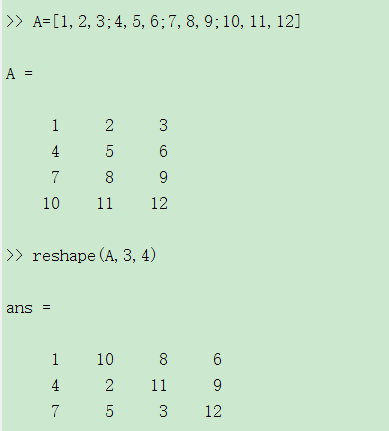查看矩阵的大小

size(A)
列出矩阵的行数和列数

size(A，1）
返回矩阵的行数

size（A,2）
返回矩阵的列数

length(A)
等价于max(size(A))

矩阵的行列式
把一个方阵看作一个行列式，并对其按行列式的规则求值，这个值就称为矩阵所对应的行列式的值。在MATLAB中，求方阵A所对应的行列式的值的函数是det(A)。
矩阵的秩与迹
矩阵的秩 矩阵线性无关的行数与列数称为矩阵的秩。在MATLAB中，求矩阵秩的函数是rank(A)。
矩阵的迹矩阵的迹等于矩阵的对角线元素之和，也等于矩阵的特征值之和。在MATLAB中，求矩阵的迹的函数是trace(A)。
向量和矩阵的范数
矩阵或向量的范数用来度量矩阵或向量在某种意义下的长度。范数有多种方法定义，其定义不同，范数值也就不同。
(1) 向量的3种常用范数及其计算函数 在MATLAB中，求向量范数的函数为：
a、norm(V)或norm(V,2)：计算向量V的2-范数；
b、norm(V,1)：计算向量V的1-范数；
c、norm(V,inf)：计算向量V的∞-范数。
(2) 矩阵的范数及其计算函数 MATLAB提供了求3种矩阵范数的函数，其函数调用格式与求向量的范数的函数完全相同。
(3) 矩阵的条件数 在MATLAB中，计算矩阵A的3种条件数的函数是：
a、cond(A,1) 计算A的1-范数下的条件数；
b、cond(A)或cond(A,2) 计算A的2-范数数下的条件数；
c、cond(A,inf) 计算A的 ∞-范数下的条件数。
矩阵的特征值与特征
向量在MATLAB中，计算矩阵A的特征值和特征向量的函数是eig(A)，常用的调用格式有3种：
(1) E=eig(A)：求矩阵A的全部特征值，构成向量E。
(2) [V,D]=eig(A)：求矩阵A的全部特征值，构成对角阵D，并求A的特征向量构成V的列向量。
(3) [V,D]=eig(A,’nobalance’)：与第2种格式类似，但第2种格式中先对A作相似变换后求矩阵A的特征值和特征向量，而格式3直接求矩阵A的特征值和特征向量。
矩阵的基本运算
矩阵的加减
对应分量进行运算，要求参与加减运算的矩阵具有 相同的维数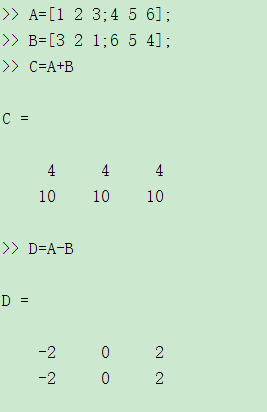矩阵的普通乘法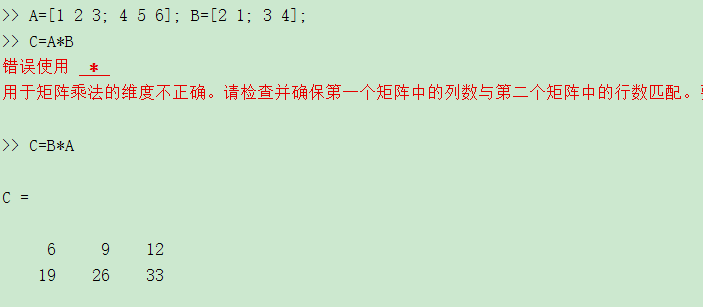矩阵的除法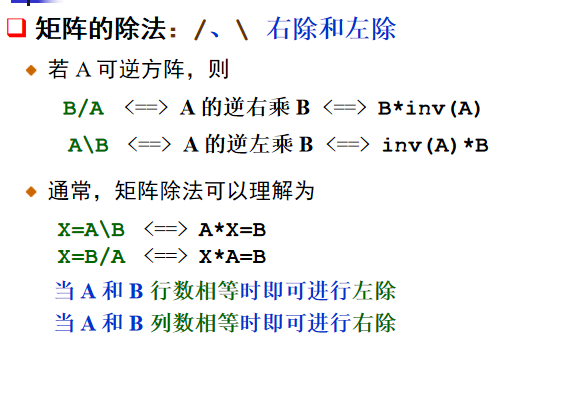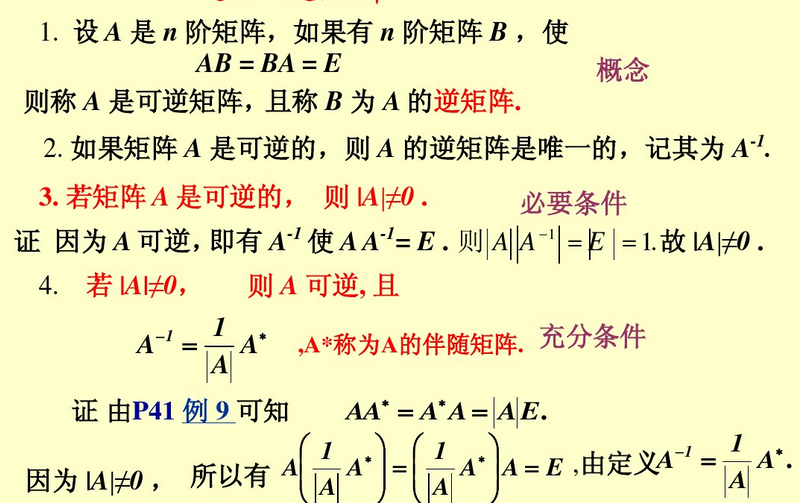矩阵的乘法
A 是方阵，p 是正整数
A^p  表示 A 的 p 次幂，即 p 个 A 相乘。
若 A 是方阵，p 不是正整数
A^p 的计算涉及到 A 的特征值分解，即若
A = VDV-1
则    Ap=V*(D.p)/V
矩阵的 Kronecker 乘积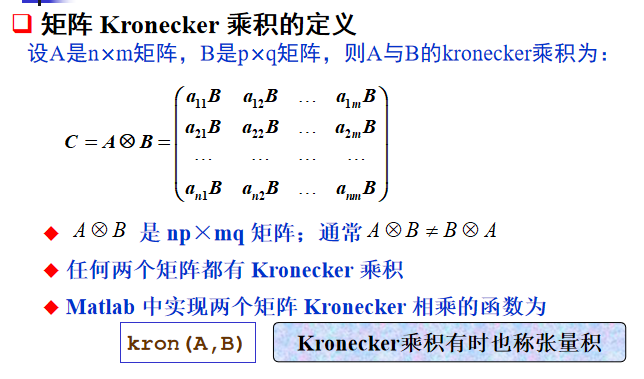矩阵的数组运算
数组运算：对应元素进行运算
数组运算包括：点乘、点除、点幂
相应的数组运算符为： “.* ” ， “./ ” ， “.\ ” 和“ .^ ”
点与算术运算符之间不能有空格！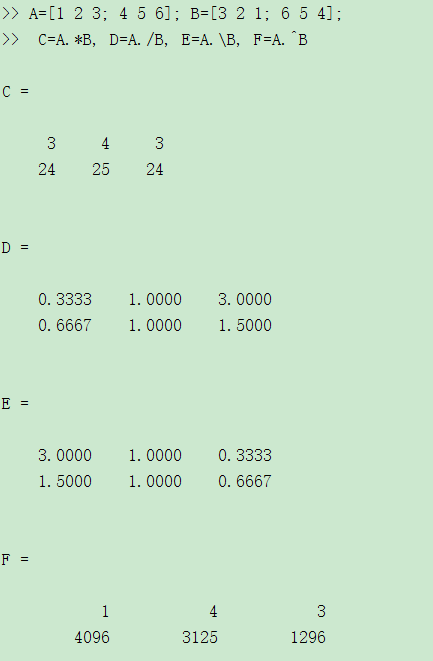矩阵中所有元素
矩阵所有元素求和

sum(A)
对矩阵每一列的元素分别求和

sum(A,2)
对矩阵每一行的元素分别求和

sum(A(😃)
将矩阵的所有元素相加求和

求期望

mean(A)
对矩阵每一列的元素分别求期望

mean(A,2)
对矩阵每一行的元素分别求期望

mean(A(😃)
将矩阵的所有元素相加求期望

求均方差
std2（A）:求矩阵所有元素的均方差
矩阵与数的运算
加减：矩阵的每个元素都与数作加减运算
数乘：矩阵的每个元素都与数作乘法运算
矩阵除以一个数：每个元素都除以这个数
点幂：
底为矩阵，指数为标量
底为标量，指数为矩阵
稀疏矩阵
MATLAB的矩阵有两种存储方式：完全存储方式和稀疏存储方式。
完全存储方式
完全存储方式是将矩阵的全部元素按列存储。以前讲到的矩阵的存储方式都是按这个方式存储的，此存储方式对稀疏矩阵也适用。
稀疏存储方式
稀疏存储方式仅存储矩阵所有的非零元素的值及其位置，即行号和列号。在MATLAB中，稀疏存储方式也是按列存储的。
注意，在讲稀疏矩阵时，有两个不同的概念，一是指矩阵的0元素较多，该矩阵是一个具有稀疏特征的矩阵，二是指采用稀疏方式存储的矩阵。
sparse(S)
将矩阵S转化为稀疏存储方式。当矩阵S已经是稀疏存储方式时，则函数调用相当于ans=S。
例如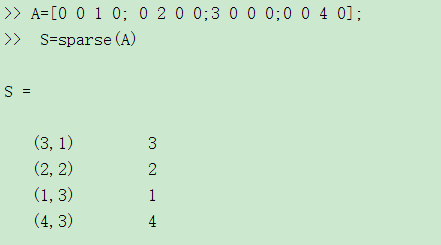sparse函数还有其他一些调用格式：sparse(m,n)：生成一个m×n的所有元素都是0的稀疏矩阵。
sparse(u,v,s,m,n)：u,v,s是3个等长的向量
s是要建立的稀疏矩阵的非0元素
u(i)、v(i)分别是s(i)的行和列标
m,n分别是矩阵的行数和列数。当m,n未被指定时，该函数建立一个max(u)行、max(v)列并以s为稀疏元素的稀疏矩阵
还有一些和稀疏矩阵操作有关的函数。例如[u,v,s]=find(A)：返回矩阵A中非0元素及下标。产生的u,v,s可作为sparse(u,v,s)的参数。full(A)：返回和稀疏存储矩阵A对应的完全存储方式矩阵。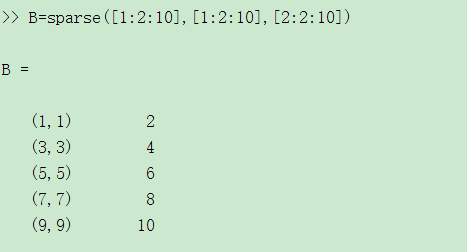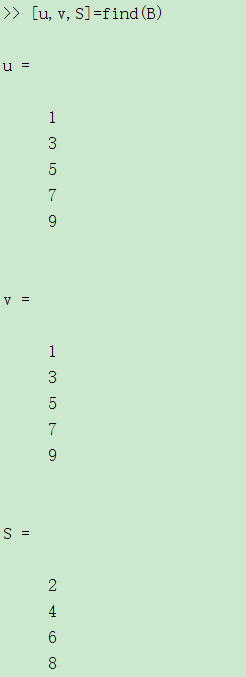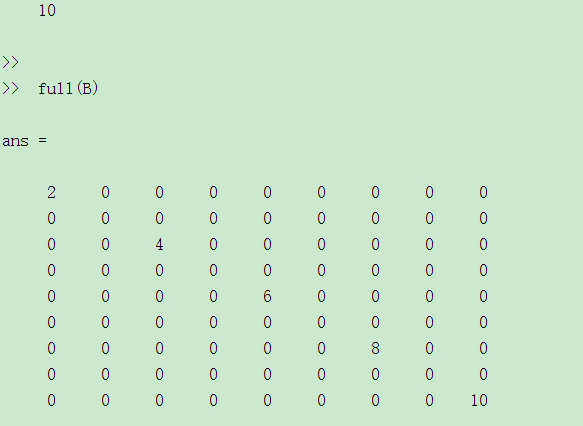特殊稀疏矩阵
单位矩阵只有对角线元素为1，其他元素都是0，是一种具有稀疏特征的矩阵。
函数eye产生一个完全存储方式的单位矩阵。
函数speye(m,n)返回一个m×n的稀疏存储方式的单位矩阵。若m=n可简写为speye(n)。
spones(S):把矩阵S的非零元素值改为1。
sprand：产生非零元素为均匀分布的随机数的稀疏矩阵 。
稀疏存储矩阵只是矩阵的存储方式不同，它的运算规则与普通矩阵是一样的。所以，在运算过程中，稀疏存储矩阵可以直接参与运算。当参与运算的对象不全是稀疏存储矩阵时，所得结果一般是完全存储形式。


展开全文• ## MATLAB定义随机矩阵

万次阅读 2017-11-15 14:50:23
A = rand(3, 5) %定义一个3行5列的随机矩阵 size(A) %返回值是3 5 rows = size(A, 1) %取到行数，1指代取行数 cols = size(A, 2) %取到列数，2指代取列数 注意：目前MATLAB中下标都是从1开始的
1，A = rand(3, 5)    %定义一个3行5列的随机矩阵（范围为0-1之间的小数）
size(A)     %返回值是3   5
rows = size(A, 1)    %取到行数，1指代上面返回值的第一个，即行数
cols = size(A, 2)    %取到列数，2指代上面返回值的第二个，即列数 注意：目前MATLAB中下标都是从1开始的
2，A = randi(7, 3, 5)   %定义一个满足均匀分布3行5列的随机矩阵（范围为1-7之间的整数）
3，A = randn(3, 5)   %定义一个满足标准正态分布的3行5列的随机矩阵
展开全文• 这里字符串矩阵（或者有更标准的名称？）是指一个矩阵中的每个元素为changdua
这里字符串矩阵（或者有更标准的名称？）是指一个矩阵中的每个元素为长短不一的字符串，如：
['hello' 'hi'; 'hello' 'world']
在未知元素字符串长度的时候，可以逐元素定义：
StrMat{m,n}='this is a string'
注意这里↑，括号是花（大）括号。


展开全文字符串
• ## MATLAB01:基本的数学运算与矩阵运算

万次阅读 多人点赞 2019-11-12 17:22:52
基本的数学运算与矩阵运算MATLAB基本语法变量变量名保留变量不适合做变量名变量不...MATLAB进行矩阵运算定义矩阵向终端输入矩阵使用冒号运算符创建向量定义特殊矩阵矩阵的索引矩阵的操作操作矩阵的运算符操作矩阵的...
• https://jingyan.baidu.com/article/3c343ff7a61b5f0d37796384.html zz2=ones(1080,1920,3)*nan 或者 zz2=zeros(1080,1920,3)*nan
• 正交矩阵：AA' = A'A = I 在matlab中生成一个随机正交矩阵
• { //如何定义M1为空矩阵 /*M1 = (int **)malloc(sizeof(int *)); for (i = 0; i ; i++) { for (j = 0; j ; j++) { *(M1 + i) = (double *)malloc(sizeof(double) * 3); } } for (i...c
• 参考：...MATLAB定义一个char型矩阵，如需要定义测站列表，测站名为四个字符，有22个测站，应该生成一个22*4的char型矩阵： 生成的site变量如下： ...
• 看论文时，经常看到矩阵，但记忆里又看到数组。那么问题来了，矩阵和数组分别是什么...数学上，定义m×n数(i=1, 2…, m ; j=1, 2,… n)排成的m行n列的数表示为m行n列的矩阵，并且用大写加粗黑色字母表示。 ...
• 在matlab中，利用for循环来生成一个矩阵，并分别对该矩阵的行和列进行求和 先创建一个新的脚本，并保存，编辑器进行编辑。具体如图，以5阶方阵为例： a=zeros（5，5）； for i=(1:5) for j=(1:5) a(i,j)=i*j; ...
• vc定义一下一个数组并生成matlab矩阵 static double real_data[]={1.1, 2.56, 3, 4, 5, 6, 7, 8, 9};mat0=mxCreateDoubleMatrix(3,3,mxREAL); memcpy((void*)mxGetPr(mat0),(void*)real_data,sizeof(real_data)); ...存储
• MATLAB 定义数组时，行数和列数必须是提前确定好的，因此，MATLAB定义矩阵一定是等宽的。 而 java 定义数组时，可以只确定行数，因此，可以定义不等宽的矩阵。 举例：  double[][] e = new double[]; for...java
• 的灰度共生矩阵相关函数用法 （ graycomatrix ， graycoprops ）   matlab 的灰度共生矩阵相关函数用法（ graycomatrix ， graycoprops ）   graycomatrix ：由图像创建灰度共生矩阵   1....
• 在MATLAB中创建矩阵有以下规则： a、矩阵元素必须”[ ]”内； b、矩阵的同行元素之间用空格或逗号隔开； c、矩阵的行与行之间用”;”（或回车符）隔开； d、矩阵的元素可以是数值、变量、表达式或函数；
• x=1:0.1:10; y=[]; for i=1:length(x) % y=[y;x(i)];%把每一个x都放到Y里，成为一列 y=[y,x(i)];%把每一个x都放到Y里，成为一行 end
• 1、定义一个矩阵  定义一个3*3的矩阵；  定义一个全为0的矩阵，使用zeros(m,n)定义一个m*n的矩阵；... 定义一个范围0-1之间的矩阵，使用rand(m,n) A = [1 2;3 4;5 6] B = zeros(3,3) C = ones(3,4) D...Octave
• 文章目录一、联系二、创建 一、联系 一维数组相当于向量,二维数组相当...矩阵一个二维数组，所以矩阵的加、减、数乘等运算与数组运算是一致的。但有两点要注意： (1)对于乘法、乘方和除法等三种运算，矩阵运算与数...
• matlab中的所有数据都按照数组的形式进行存储和运算，数组的属性和数组之间的逻辑关系是编写程序时非常重要的两方面。在matlab平台上，数组的定义是广义的，数组的元素可以是任意的数据类型，例如可以是数值、字符...数组
•   C语言，我们已经学过种变量定义形式——结构体，其使用方法可如下： 需要.h头文件声明结构体： struct 结构体名 { 数据类型 变量名1； };   其中的数据类型既可以是约定好的int、char、float等...
• 在MATLAB中创建矩阵有以下规则： a、矩阵元素必须”[ ]”内； b、矩阵的同行元素之间用空格（或”,”）隔开； c、矩阵的行与行之间用”;”（或回车符）隔开； d、矩阵的元素可以是数值、变量、表达式...
• matlab中矩阵维数不一致，可能是之前定义一个矩阵，后来又定义一个同名矩阵，2个矩阵维数不同，混用一起了。 还可能矩阵未定义。
• ## Matlab中的矩阵运算

千次阅读 2014-05-08 21:20:14
利用Matlab函数创建矩阵 利用文件创建矩阵 二、矩阵的拆分 矩阵元素 矩阵拆分 特殊矩阵 三、矩阵的运算 算术运算 关系运算 逻辑运算 四、矩阵分析 对角阵 三角阵...matrix math
• 因为矩阵一个数学概念（线性代数里的），数组是个计算机上的概念。 《精通MATLAB6.5版》（张志涌编著，北京航空航天大学出版社）说： 从外观形状和数据结构上看，二维数组和数学矩阵没有区别。但是矩阵作为...
• 所有MATLAB中矩阵数据都按列存放。 矩阵的建立 1.直接输入法  * 将矩阵的元素用方括号括起来，按矩阵行的顺序输入各元素，同一行的各元素之间用空格或逗号分隔，不同行的元素之间用分号分隔。  * 矩阵...
• matlab中如何提取矩阵中指定位置元素 1）指定行和列:A(m,n),提取第m行，第n列 2）某一行全部元素：A(m,:) 3）某一列全部元素：A(:,n) 4) 取出某些行：A([m,n],: )（第m行和第n行组成一个矩阵） 5）取出某行到某行：A...
• ## Matlab矩阵运算

千次阅读 2014-09-11 10:52:20
说明：这段时间用Matlab做了LDPC码的性能仿真，过程涉及了大量的矩阵运算，本文记录了Matlab中矩阵的相关知识，特别的说明了稀疏矩阵和有限域矩阵Matlab的运算是在矩阵意义下进行的，这里所提到的是狭义上...
• 在Matlab当中，我们可以采用图形界面的lmiedit命令，来调用GUI接口，但是我认为采用程序的方式更方便（也因为我不懂这lmiedit的GUI）。 对于LMI Lab， 其中有三种求解器（solver）： feasp，mincx和gevp。 每......

# 如何在matlab中定义一个矩阵matlab 订阅